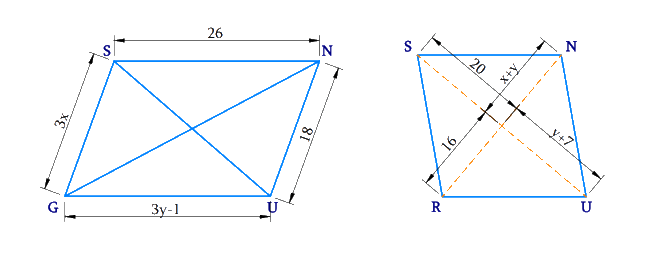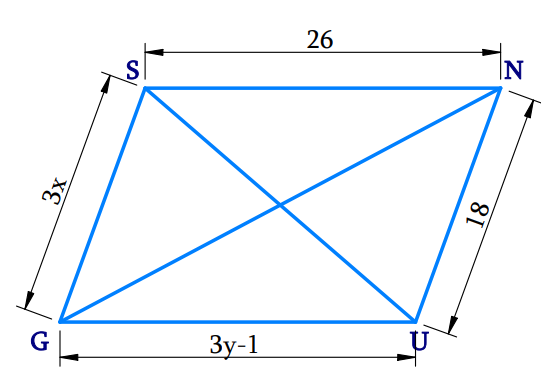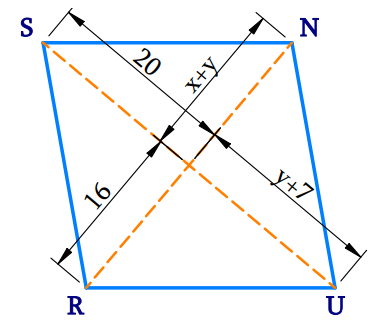# Ex.3.3 Q8 Understanding Quadrilaterals Solution-Ncert Maths Class 8

Go back to  'Ex.3.3'

## Question

The following figures $$GUNS$$ and $$RUNS$$ are parallelograms. Find $$x$$ and $$y$$. (Lengths are in $$\rm cm$$)Video Solution
Ex 3.3 | Question 8

## Text Solution

i) What is Known?

Given figure is a parallelogram.

What is Unknown?

Values of $$x$$, $$y$$

Reasoning:

The diagonals of a parallelogram bisect each other, in a parallelogram, the opposite sides have same length.

Steps:In a parallelogram, the opposite sides have same length.

\begin{align}{\rm{SG}}\,\rm&= \,{\rm{NU}}\\3x &= 18\\x &= \,\frac{{18}}{3}\\x &= 6\end{align}

And,

\begin{align}\text{SN}&=\text{GU} \\ 26&=3y-1 \\ 3y&=26+1 \\y&=\frac{27}{3} \\y&=9 \\\end{align}

Hence, the measures of $$x$$ and $$y$$ are $$6 \rm\, cm$$ and $$9 \rm \,cm$$ respectively.

(ii) What is Known?

Given figure is a parallelogram.

What is Unknown?

Values of $$x, y$$

Reasoning:

The diagonals of a parallelogram bisect each other. In a parallelogram, the opposite sides have same length.

Steps:Property: The diagonals of a parallelogram bisect each other.

\begin{align}y{\rm{ }} + {\rm{ }}7{\rm{ }} &= {\rm{ }}20\\y &= 20 - 7\\y{\rm{ }} &= {\rm{ }}13\\ x{\rm{ }} + {\rm{ }}y{\rm{ }} &= {\rm{ }}16\\x{\rm{ }} + {\rm{ }}13{\rm{ }} &= {\rm{ }}16\\x{\rm{ }} &= {\rm{ }}3\end{align}

Hence, the measures of $$x$$ and $$y$$ are $$3 \rm\,cm$$ and $$13 \rm\,cm$$ respectively.

Learn from the best math teachers and top your exams

• Live one on one classroom and doubt clearing
• Practice worksheets in and after class for conceptual clarity
• Personalized curriculum to keep up with school